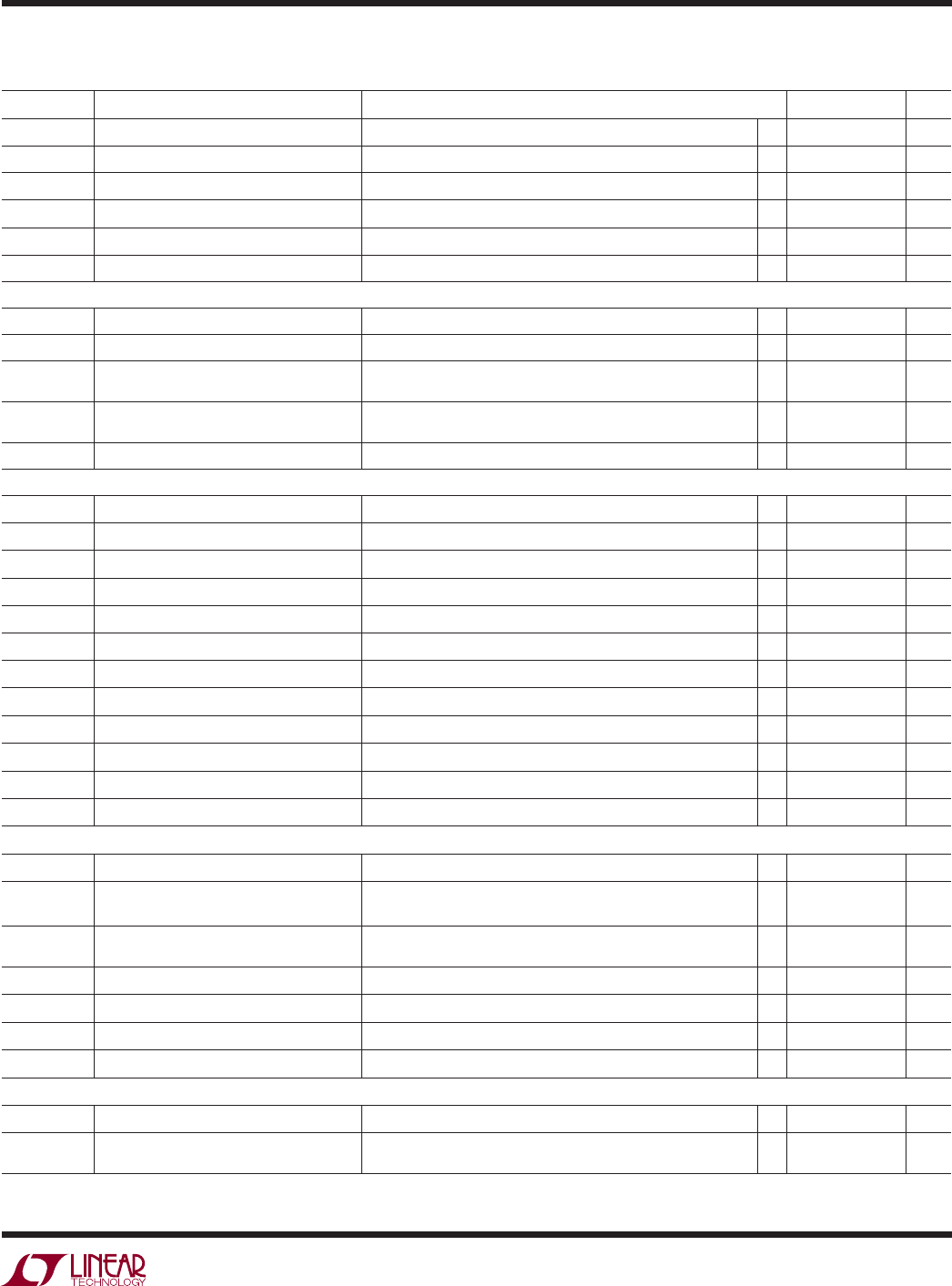# DatasheetLTC4309
3
4309fa
The l denotes the speciﬁ cations which apply over the full operating
temperature range, otherwise speciﬁ cations are at T
A
= 25°C. V
CC
= 3.3V, V
CC2
= 3.3V, unless otherwise noted.
SYMBOL PARAMETER CONDITIONS MIN TYP MAX UNITS
V
CC
Positive Supply Voltage
l
2.3 5.5 V
V
CC2
Input Side Accelerator Supply Voltage
l
1.8 5.5 V
I
CC
V
CC
Input Supply Current Enabled V
CC
= V
CC2
= 5.5V, V
SDAIN
= V
SCLIN
= 0V (Note 2)
l
711 mA
I
SD
V
CC
Input Supply Current Disabled V
CC
= V
CC2
= 5.5V, SDA = SCL = 5.5V, ENABLE = OV
l
900 1400 μA
I
CC2
V
CC2
Input Supply Current Enabled V
CC
= V
CC2
= 5.5V, V
SDAIN
= V
SCLIN
= 0V (Note 2)
l
190 250 μA
I
SD2
V
CC2
Input Supply Current Disabled V
CC
= V
CC2
= 5.5V, SDA = SCL = 5.5V, ENABLE = OV
l
140 180 μA
Propagation Delay and Rise Time Accelerators
t
PHL
SDA/SCL Propagation Delay High to Low C
= 50pF, 2.7k to V
CC
on SDA, SCL, (Note 3, 4), (Figure 1) 85 ns
t
PLH
SDA/SCL Propagation Delay Low to High C
= 50pF, 2.7k to V
CC
on SDA, SCL, (Note 3, 4), (Figure 1) 10 ns
t
RISE
SDA/SCL Rise Time C
= 100pF, 10k to V
CC
on SDA, SCL, V
CC
= 5V V
CC2
= 5V,
(Note 3, 5), (Figure 1)
30 300 ns
t
FALL
SDA/SCL Fall Time C
= 100pF, 10k to V
CC
on SDA, SCL, V
CC
= 5V (Note 3, 5),
(Figure 1)
30 300 ns
I
PULLUPAC
Transient Boosted Pull-up Current Positive Transition > 0.8V/μS on SDA, SCL, V
CC
= 3.3V (Note 7) 5 8 mA
Start-Up Circuitry
V
PRE
Precharge Voltage SDA, SCL Open
l
0.8 1.0 1.2 V
t
IDLE
Bus Idle Time
l
55 95 175 μs
V
THR_EN
ENABLE Threshold Voltage ENABLE Rising Edge
l
0.8 1.4 2 V
V
THR_EN(HYST)
ENABLE Threshold Voltage Hysteresis (Note 3) 100 mV
V
THR_CTRL
ACC, DISCEN Threshold Voltage 0.5 0.7 1 V
I
CTRL
ENABLE, ACC, DISCEN Input Currents ENABLE, ACC, DISCEN from 0 to V
CC
l
0.1 ±5 μA
t
PLH_EN
ENABLE Delay Off-On (Figure 1) 95 μs
t
PHL_EN
ENABLE Delay On-Off (Note 3), (Figure 1) 10 ns
t
READY Delay On-Off (Note 3), (Figure 1) 10 ns
t
READY Delay Off-On (Note 3), (Figure 1) 10 ns
V
= 3mA, V
CC
= 2.3V
l
0.4 V
I
CC
l
0.1 ±5 μA
Timing Characteristics
f
I2C, MAX
I
2
C Maximum Operating Frequency (Note 3) 400 600 kHz
t
BUF
Bus Free Time Between Stop and Start
Condition
(Note 3) 1.3 μs
t
HD, STA
Hold Time After (Repeated)
Start Condition
(Note 3) 100 ns
t
SU, STA
Repeated Start Condition Set-Up Time (Note 3) 0 ns
t
SU, STO
Stop Condition Set-Up Time (Note 3) 0 ns
t
HD, DATI
Data Hold Time Input (Note 3) 0 ns
t
SU, DAT
Data Set-Up Time (Note 3) 100 ns
Input-Output Connection
V
OS
Input-Output Offset Voltage 2.7k to V
CC2
on SDA, SCL, Driven SDA, SCL = 0.2V
l
20 60 100 mV
V
THR
SDA, SCL Logic Input Threshold Voltage V
CC
≥ 2.9V
V
CC
< 2.9V
1.4
1.1
1.65
1.35
1.9
1.6
V
V
ELECTRICAL CHARACTERISTICS